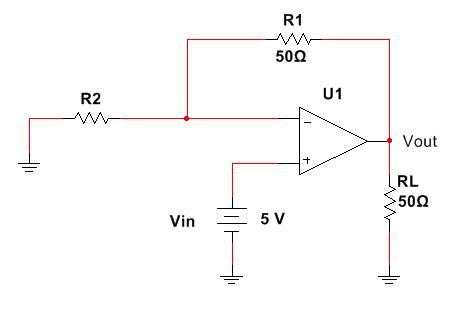# Op-Amp Circuit - Find R

• Engineering
hogrampage

## Homework Statement

Calculate the value of R2 required to deliver 5W to RL if Vin equals 5V.Vout=iRL
P=Ri^2

## The Attempt at a Solution

I started by using KVL for the input loop, noting that current is going counter-clockwise:

Input loop: iR2-Vin=0→R2=Vin/i

I found i by using P=Ri^2:

i=sqrt(5/50)
=0.316A

Then, I used i from above to solve for R2:

R2=Vin/i
=5/0.316
=15.8Ω

The answer should be 23.1Ω according to the book (Engineering Circuit Analysis 8ed). Where did I go wrong? I have tried so many different approaches, but I can never get to the answer of 23.1Ω.

Staff Emeritus
Homework Helper
The currents through R2 and RL are not the same.

Question for you: what must Vout be, to get the necessary power in RL?

hogrampage
Wow, how did I not see the currents were not the same?

Anyway, I figured it out after finding Vout (15.81V). It makes complete sense to me now, thank you so much for your help!

Staff Emeritus